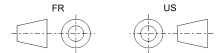# Orthographic projection

For the orthographic projection as a map projection, see Orthographic projection in cartography.
For mathematical discussion in terms of linear algebra, see Projection (linear algebra).

Orthographic projection (or orthogonal projection) is a means of representing a three-dimensional object in two dimensions. It is a form of parallel projection, where all the projection lines are orthogonal to the projection plane, resulting in every plane of the scene appearing in affine transformation on the viewing surface. A lens providing an orthographic projection is known as an (object-space) telecentric lens.

The term orthographic is also sometimes reserved specifically for depictions of objects where the axis or plane of the object is also parallel with the projection plane, as in multiview orthographic projections.

## Origin

The orthographic projection has been known since antiquity, with its cartographic uses being well documented. Hipparchus used the projection in the 2nd century BC to determine the places of star-rise and star-set. In about 14 BC, Roman engineer Marcus Vitruvius Pollio used the projection to construct sundials and to compute sun positions.

Vitruvius also seems to have devised the term orthographic (from the Greek orthos (= “straight”) and graphē (= “drawing”) for the projection. However, the name analemma, which also meant a sundial showing latitude and longitude, was the common name until François d'Aguilon of Antwerp promoted its present name in 1613.

The earliest surviving maps on the projection appear as woodcut drawings of terrestrial globes of 1509 (anonymous), 1533 and 1551 (Johannes Schöner), and 1524 and 1551 (Apian).

## Geometry

A simple orthographic projection onto the plane z = 0 can be defined by the following matrix:For each point v = (vx, vy, vz), the transformed point would beOften, it is more useful to use homogeneous coordinates. The transformation above can be represented for homogeneous coordinates asFor each homogeneous vector v = (vx, vy, vz, 1), the transformed vector would beIn computer graphics, one of the most common matrices used for orthographic projection can be defined by a 6-tuple, (left, right, bottom, top, near, far), which defines the clipping planes. These planes form a box with the minimum corner at (left, bottom, -near) and the maximum corner at (right, top, -far).

The box is translated so that its center is at the origin, then it is scaled to the unit cube which is defined by having a minimum corner at (-1,-1,-1) and a maximum corner at (1,1,1).

The orthographic transform can be given by the following matrix:which can be given as a scaling followed by a translation of the formThe inversion of the Projection Matrix, which can be used as the Unprojection Matrix is defined:## Multiview orthographic projectionsSymbols used to define whether a projection is either Third Angle (right) or First Angle (left).

With multiview orthographic projections, up to six pictures of an object are produced, with each projection plane parallel to one of the coordinate axes of the object. The views are positioned relative to each other according to either of two schemes: first-angle or third-angle projection. In each, the appearances of views may be thought of as being projected onto planes that form a 6-sided box around the object. Although six different sides can be drawn, usually three views of a drawing give enough information to make a 3D object. These views are known as front view, top view and end view.

## Cartography

An orthographic projection map is a map projection of cartography. Like the stereographic projection and gnomonic projection, orthographic projection is a perspective (or azimuthal) projection, in which the sphere is projected onto a tangent plane or secant plane. The point of perspective for the orthographic projection is at infinite distance. It depicts a hemisphere of the globe as it appears from outer space, where the horizon is a great circle. The shapes and areas are distorted, particularly near the edges.Wikimedia Commons has media related to Orthographic projections.